info@biomedres.us   +1 (720) 414-3554
One Westbrook Corporate Center, Suite 300, Westchester, IL 60154, USA

# Biomedical Journal of Scientific & Technical Research

September, 2021; Volume 38, 5; pp 30661-30670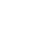Research Article

Research Article

# Fast GPU Interpolation for Medical Robotics Using the Conformal Geometric Algebra Framework

### Gerardo Martinez Teran, Oswaldo Urena-Ponce, Gerardo Soria Garcia, S Ortega-Cisneros and Eduardo Bayro Corrochano*

Author Affiliations

Department of Electrical Engineering and Computer Science, Mexico

Received: September 01, 2021 | Published: September 15, 2021

Corresponding author: Eduardo Bayro Corrochano, Department of Electrical Engineering and Computer Science, Mexico

DOI: 10.26717/BJSTR.2021.38.006201

#### ABSTRACT

Interpolating trajectories of points and geometric entities is an im- portant problem for kinematics. To describe these trajectories, several algorithms have been proposed using matrices, quaternions, dual-quaternions, and the Study quadric; the last one allows the embedding of motors as 8D vectors into projective space P7, where the interpolation of rotations and translations becomes a linear problem. Furthermore, conformal geometric algebra (CGA) is an effective and intuitive framework for representing and manipulating geometric entities in Euclidean spaces, and it allows the use of quaternions and dual-quaternions for- mulated as Motors. In this paper, a new methodology for accelerating the Study quadric Interpolation based on Conformal Geometric Algebra is presented. This methodology uses General Purpose Graphics Processing Units (GPUs) and it is applied for medical robotics, but it can be also extended to other areas like aeronautics, robotics, and graphics processing.

Keywords: Motor Interpolation; Conformal Geometric Algebra; Motor Algebra

#### Introduction

Generating trajectories for 3D objects is a fundamental problem in areas of robotics, image processing, and others. There are few mathematical algorithms for the interpolation of points that use vector calculus, quaternions, dual-quaternions, and linear algebra. Geometric algebra is a powerful mathematical framework for solving problems using basic geometry entities (circles, points, spheres, planes, and lines), and can represent Euclidean geometry, quaternions, and dual quaternions. For this reason, it can be used for interpolating geometric entities for applications in medical robotics, graphic engineering, robotics, and aeronautics. In this work, a GPU implementation for interpolating geometric entities of conformal geometric algebra is proposed. The design is based on an interpolation algorithm that maps rotations and translations of motors as 8D vectors in the Study manifold.

The paper is organized as follows: section ?? reports on related work, section 2 gives a brief introduction to geometric algebra, with special emphasis on conformal geometric algebra. Section 3 presents the motor interpolation algorithm based on geometric algebra for interpolating entities in different dimensions, while section 4 describes the optimization and implementation of the interpolation algorithm in GPU and section 5 shows the experimental results. Finally, section 7 presents our conclusions and future work. Due to the easy representation of complex problems in robotics and image processing, several works have been proposed to accelerate geometric algebra operations. Most related techniques for accelerating geometric algebra algorithms are based on speeding up basic operations (inner, outer and geometric product, etc) with FPGA Co-processors [1-4] and GPU parallelization [5,6]. More complex architectures have been developed for applications on computational vision. In  the authors present an algorithm of Clifford convolution and Clifford Fourier transforms for color edge detection, and an alternative algorithm based on rotor edge detection is proposed in .

Gerardo Soria-Garc´ıa et al. introduce an FPGA implementation of Conformal Geometric Algebra Voting Scheme for Geometric Entities Extraction, such as lines, and circles on images of edges . A GPU implementation for conformal geometric algebra interpolation application based on GPU is presented in . The algorithm presented in this work is a modification using motors of the dual-quaternion method to interpolate rotation, translation, and dilation of the geometric entities like points, lines, circles, pair of points, planes, and spheres.

#### Geometric Algebra

In an n-dimensional real vector space Rn, we can introduce an orthonormal basis of vectors { }, i = 1, ..., n. This leads to a basis for the entire geometric algebra.The Geometric Algebra (GA) of the real n-dimensional quadratic vector space (V, Q) is denoted as Gn. In the case Gp,q,r (n = p + q + r) denotes the GA of (V, Q) where p, q, r correspond the number of the basis vectors which square in GA to 1,-1,and 0, respectively. The geometric product of two vectors a and b is written as ab and can be expressed as a sum of its symmetric and antisymmetric parts: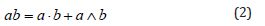where the inner product a · b and the outer product a ∧ b is defined by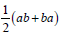andGn = Gp,q,r when n = p + q + r with p-unit vectors, q-unit vectors and r-unit vectors which square to 1,-1 and zero respectively. Gn is a graded linear space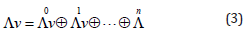where the elements of ∧n are referred to as (homogeneous) multivectors of grad k for k = 0,1, 2,…..,n. In the following, for short, elements of ∧1 V are called vectors. Thus, any M ∈ Gn can be uniquely decomposed into a sum Σk < M >k where < M >k∈ ∧k V. Furthermore, Gn is a Z2-graded algebra in the following sense: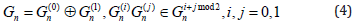WhereThen,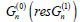is called the even (resp. the odd) part pf Gn. Note that due to equation (4),is a subalgebra of Gn. Later in this paper, the even subalgebra of Gn will be denoted by .

The dimension of Gn is 2n. The multivector basis of Gn has 2n bases for scalars, bivectors, trivectors and k-vectors. A k-blade is either the identity element 1 of Gn (when k = 0 or, when k > 0), it is defined as the wedge productA linear combination of k-vectors is called a homogeneous multivector. Consider two homogeneous multivectors Ar and Bs ∈ G n of grades r and s, respectively. The geometric product of Ar and Bs can be written as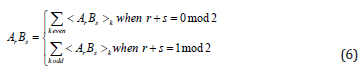### Conformal Geometric Algebra

In Conformal Geometric Algebra G4,1 (CGA), the Euclidean vector space R3 is represented in R4,1. The space for G4,1 has an orthonormal vector basis given by {e1, e2, e2, e4, e5} with the properties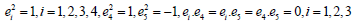The null basis {e0 ,e∞ } (origin and point at infinity) is defined as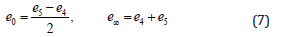These null vectors satisfy the relations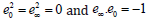Let be E = e∞ ∧ e 0 the Minkowski plane. The unit Euclidean pseudo-scalar is Ie:= e1∧e2∧e3, and the conformal pseudoscalar Ic = Ie E is used for computing the inverse and duals of multivectors. Given a nonsingular k-vector Ak ∈ G4,1 ≡ G4,1,0, the dual and its inverse are respectively.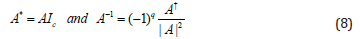where A+ stands for the reversion of A and |A| its magnitude. (Table 1) where IPNS and OPNS stand for Inner Product Null Space and Outer Product Null Space respectively.

Table 1: Representation of entities in conformal geometric algebra.

### Points, Lines, Planes, and Spheres

The representation of a 3D Euclidean point x = [ x, y, z ]T in R3 in the geometric algebra G4,1 is given byGiven two conformal points xc and yc, their difference in Euclidean space can be defined as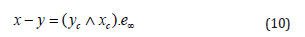and, consequently, the following equality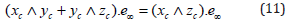is fulfilled as well. The line is formulated as a form in the Inner Product Null Space (IPNS) as followswhere n (bivector) is the orientation and the vector m the moment of the line. The plane is formulated as a form in IPNS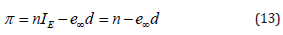where the n (bivector) for the plane orientation and d is the distance from origin orthogonal to the plane.

The sphere is formulated as a form in IPNS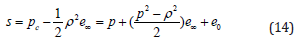A poin is a sphere with zero radius. Considering the dual equation for the sphere, we can write the constraint for a point lying on a sphere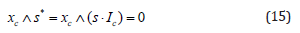The sphere can be directly computed by the wedge of four conformal points in the Outer Product Null Space (OPNS)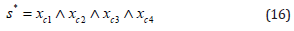Replacing any of these points with the point at infinity, we obtain the OPNS plane equation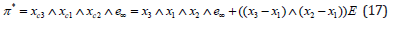Similar to Eq. (17), the OPNS line equation can be formulated as a circle passing through the point at infinity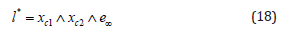### Rigid Transformations

In CGA many of the transformations can be formulated in terms of successive reflections between planes.

Reflection: The equation of a point x reflected with respect to a plane π is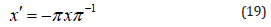Translation: The transformation of geometric entity O formulated as two successive reflections w.r.t. the parallel planes π1 and π2 is given by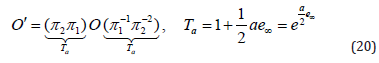where a = 2dn, d is the distance of translation, and n is the direction of translation

Rotation: Similarly, we can formulate a rotation as the product of two reflections between non-parallel planes π1 and π2 which cross the origin.The geometric product of the unit normal vectors of these planes n1 and n2 yields the equation for the rotor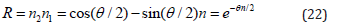where the unit bivector n = n1 ∧n2, and teh angle θ is twice the angle between π1 and π2

Screw Motion: The operator for screw motion or motor M is a composition of a translator T and a rotor R, both w.r.t. to an arbitrary axis L. The equation for a motor is as follows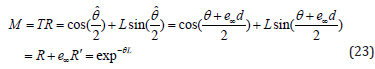where the screw line is n and m stand for the orientation and momentum of the screw line and the dual angle angle . Finally, the motor transformation for any object O ∈ G4,1 reads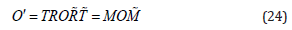#### Interpolation Algorithm Using the Study Quadric Manifold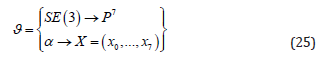where SE (3) is the special Lie group for 3D Euclidean rigid transformations, α represents any Euclidean rigid transformation and the vector X containing homogeneous coordinates X ∈ M6. Note that the motor algebra is a sub-algebra of the 3D conformal geometric algebra G4,1, thus the interpolation uses motors depending upon which algebra is used one can use I for motors of or e∞ for motors of G4,1 which both square to zero and are acting similarly as the isotropic operator of the dual quaternions which use ε which squares to zero as well. Given the set X ∈ M6 containing three homogeneous points X1, X2, X3 ∈ P7, the interpolation curve X ∈ M6 is generated by interpolating the given homogeneous points X1, X2, Xn ∈ P7which satisfy the set X computed as follows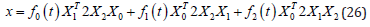The interpolation polynomials f0(t), f1(t), and f2(t) are formulated as follows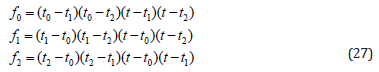where t, t0, t1, and t2 are interpolation values between 0 and 1. t represents the segment of curve where the point is calculated, t0, t1 and t2 are values that represent the section of curve where interpolated and control points meeting. The Study-quadric bilinear form is given by the following matrix G,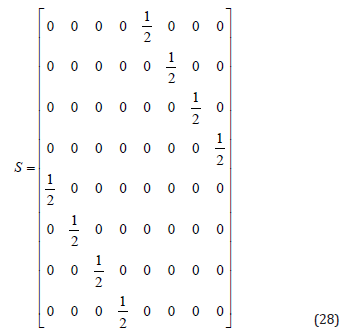If one wishes to interpolate more points, the above-explained interpolation equation can be extended and adapted as De Casteljou algorithm . This is shown on Algorithm 1. This algorithm calculates a homogeneous point for the given set X that contains the control points (X1,··· , XN) and the interpolation values (t, t0,t1,t2) and the the Study quadric bilinear matrix Q. Here t, t0, t1, t2 are interpolation variables. t is the position interval variable and allows to determine the position within the curve of the interpolation point, the value t goes from 0 to 1. To calculate all the points of the curve, all the values of t are traversed. (t0, t1,t2) are values that indicate at which point the interpolation points, in the functions that came in Klawiter library , the values t0 = 0,t1 = 0.5,t2 = 1 are proposed so that the interpolated points correspond to the initial control point, the mid-control point and the endpoint respectively. These are fixed for each curve, only t is what can change when calculating a point within a specific curve.

First, the algorithm checks the number of elements in X. If there is only one point, this is returned as the solution. Otherwise, X is used to get intermediate control points via equation (26) and saved in M. M is used to call the algorithm recurrently until we get only one interpolation point and returned it. Figure 1 shows the algorithm interpolation for five control points. See that in each interpolation step, for N control points, we get N−2 interpolation points and N −1 repeats are required. Since in the last step, equation (26) required 3 points, an odd number of control points are needed. where X is the set of control homogeneous points (X1, ·, XN) that describe the curve trajectory, G is the quadric matrix, t0, t1, and t2 are global constant values, in this case,0, 0.5, and 1 respectively. where G is the quadric matrix, and X the array of homogeneous points that contains the points X1 to Xn, t0, t1, and t2 are constant values, in this case, 0.5, and 1 respectively. As is seen int the algorithm, is required that the array X have an odd number of homogeneous points to get an interpolation curve. Note that the solution is in fact a rational motion utilizing a interpolating spline which in turn involves rational sub-spline motions, see .

Figure 1: Interpolation of five control points with algorithm 1.

In general, the Study quadric interpolation algorithm uses homogeneous points represented by dual-quaternions or by homogenous matrices. As we know, motors are isomorphic to dualquaternions and a motor can be represented as a homogeneous point as well. This motor represented as a vector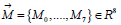Lies on the Study Manifold, see Table 2. In this regard, we propose a modified interpolation algorithm. For that, the homogeneous points are replaced by motors and substitute matrix operations with GA operations. Since the matrix multiplications in (26) with form , which result is a constant value expressed as:

Table 2: Study quadric homogeneous point represented in dualquaternion and its equivalences as motor in G+3,0,1 and as motor in G4,1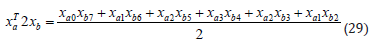Changing the homogeneous points with their respective motor representation in CGA and analyzing the motor multiplication, is shown that the coefficient of the blade e123∞ (α3) from the motor multiplication is the double value of (29). This coefficient (α3) can be extracted from any motor using the partial derivative:or via inner product with the Ie and e0: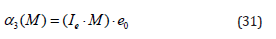Using GA, the equation (26) is reformulated for a motor based interpolation algorithm. It uses 3 control motors as follows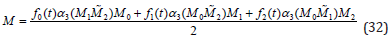In the same manner as Algorithm 1, for more control points, equation (32) can be rewritten using the De Casteljou algorithm as described on Algorithm 2. This algorithm calculates one motor gave the set M of control motors (m1,··· ,mN) and the interpolation constants (t,t0,t1,t2). Similar to Algorithm 1, the interpolation function is called recursively with the calculated motors from the last step as input until we get only one motor. Since the last step needs three motors to calculate the last one, M must contain an odd number of motors. (Algorithm 2)

Algorithm 2: Motor Interpolation on CGA.

#### Speeding up the Algorithms Using a GPU Accelerator

The algorithmic complexity using the framework Gn ∈ R 2n is: for binary operations O(22N); for unary operations O(2N), and for M interpolated motors using K motors O(MK). To accelerate the motor interpolation algorithm for real-time applications, one can utilize arithmetic accelerators based on FPGA, ASIC, and GPU. We utilize an accelerator based on CPU-GPU co-design, where the GPU is the GTX-970 by Nvidia, which has a computing capability of 5.2 and it can be implemented on any GPU using CUDA. The algorithm was developed to compute in parallel single or multiple interpolation curves. For the computing of a single interpolation curve, the default GPU was implemented, where each motor is computed in one thread launched in a Stream. For the case of multiple curves, the multi-streaming implementation was selected to overlap the streams. GTX-970 GPU does not support recursive algorithms, due to hardware limitations. To implement the motor interpolation, we translated the Algorithm 2 into an equivalent-iterative version given by the Algorithm 3.

Algorithm 3: Motor Interpolation on CGA (iterative).

The versatile Compute Unified Device Architecture (CUDA), is a platform for parallel computing, which was developed by NVIDIA for highly parallel implementations and uses its GPUs. CUDA is based on C/C++ programming languages, however other languages like Python or Fortran can be used as well. Our accelerator was written with CUDA C++ for the GPU acceleration and C++ for the CPU interface. The GTX-970 GPU does not support recursive algorithms; thus the Algorithm 2 has to be translated into an equivalent iterative version as presented in Algorithm 3. In this regard, the algorithm uses one stream for one curve of interpolation and multi-stream for multiple curves. Here, the GPU global memory is the largest in GPU, it has the highest latency, and it can be accessed from any stream for multi-stream applications. This is very helpful, if the number of motors to be computed is large, or if we compute multiple curves, or launch many algorithms employing the same GPU via multistreaming. The GPU and CPU have different architectures.
The CPU has a few sets of cores for sequential applications. In contrast, the GPU has many cores for highly parallel computing. Figure 2 compares the CPU and GPU architectures. Note that GPU cores run asynchronously using threads. Each core is limited to 32, 64, 128, 256, or 512 threads. If a parallel GPU implementation is launched, it is required a certain number of cores. These are figured out as a direct function of the selected threads per block. In the case of the motor interpolation, each thread launches a copy of the algorithm.
All GPU operations run explicitly or implicitly on a stream. If a kernel is launched in GPU, it runs explicitly or implicitly on a stream. A stream is a sequence of asynchronous operations which are executed on a device following an order ruled by the host code. A stream involves these operations to maintain order, to permit operations to be queued in the stream, and to be executed after all preceding operations. It cares for querying the status of queued operations. These operations include data transfer and kernel launches. Recent GPUs allow a parallel technique known as multi-streaming, where multiple streams are launched, and the transference data and kernel launch can be overlapped as shown in Figure 3. For the case of parallel calculation of multiple curves, the multi-streaming implementation is used, and each curve is computed in an independent stream.

Figure 2: Architecture comparison of
a) CPU and
b) GPU.

Figure 3: Different streams used for a concurrent implementa- tion
a) 1-stream,
b) Concurrent approach for device-to-host asynchronous memory copy,
c) Concurrent approach for host-to-device asynchronous memory copy,
d) Concurrent approach for host-to- device and device-to-host asynchronous memory copy.

#### Experiments

The Study’s quadric and the motor interpolation are compared to analyze which of the algorithms has the best speed performance. Many motors were calculated using 15 test groups of 5 motors considering following amount of points 20, 50, 100, 200, 500, 1000, 2000, 5000, 10000, and 20000. The test was programmed using MATLAB on a Desktop with Intel core I7 microprocessor at 2.4 GHz, 32 GB of RAM, and a GTX-970 GPU by NVIDIA. The results are presented in Figure 4. The execution time of both algorithms grew linearly while the number of points increases, thus the algorithm complexity is O(n). We see that the motor interpolation version is faster than the Study quadric interpolation, namely for 20000 points, it is 28× times faster. Figure 4 shows that the motor interpolation algorithm selected for sequential and parallel implementation utilizes same hardware parameters. The sequential implementation was programmed using C++ language and CUDA C++ for the parallel approach using 32 threads per block in the kernel. The interpolated motors were computed using single and double-precision floating-point representation. All the measures consider only the algorithm execution, but data transference, file writing, and reading are excluded. Results are shown in Figure 5.

Figure 4: Comparison of Study’s quadric and Motor interpola tion speed-up.

Figure 5: CPU and GPU speed-up analysis.

It was confirmed that the GPU has the best time performance for calculating the interpolation algorithm. When the time execution in CPU increases linearly, the time in GPU for 500 or fewer points remains almost constant. For the case of 20000 motors, the GPU implementation runs 79× and 60× times faster than the CPU for single and double floating-point respectively. The different multi-streaming implementations were analyzed; 16 different interpolation curves were calculated using the concurrent approach for host-to-device and device-to-host asynchronous memory copy with 4 configurations (1, 2, 4, and 8 streams) with 32 threads per block. The measure times include data transference. Figure 6 depicts that the use of multi-streaming reduces the execution time considerably, i.e. for 20000 points, 8 streams version is 2.7×, 1.9× and 1.6× times faster than 1, 2 and 4 streams respectively.

Figure 6: Comparison of the Multi-Stream speed-up.

#### Interpolation of Surgery Motion

This Study’s quadric-based motor interpolation was used to interpolate trajectories in medical robotics for kidney surgery, see Figure 7. The robot manipulator hast to follow a path for suture around a certain region on the kidney phantom. We design to tasks follow an Ultrasound (US)-probe trajectory and the interpolation of a given 3D points sequence. One gives the point coordinates and their desired orientations, these are interpolated by the motor algorithm, see Figures 7b-7d. Afterward, we check the precision error using inverse kinematics. The inverse kinematics was also formulated in conformal geometric algebra, the algorithm was reported in [14 -17].

Figure 7:

a) Robot; views:
b) X-Y,
c) X-Z,
d) Y-Z.

#### Conclusion

In this work, an optimized version of the Study quadric interpolation algorithm is presented using the conformal geometric algebra framework. A Matlab implementation confirms that conformal geometric algebra interpolation is 28x times faster than a Study quadric interpolation for 20000 motors. The performance of CPU and GPU approaches of motor interpolation algorithm were contrasted for the cases of single and double floating-point precision. The comparison shows that the GPU is 79x and 60 times faster than the CPU implementation for single and double precision respectively. Furthermore, 16 interpolation curves were calculated via multi-streaming. These results confirm that multi streaming reduces the execution time for multiple curves interpolation. We show an application of the motor algorithm for suture by kidney surgery. In near future, we will pursue to implement the interpolation algorithm using multiple GPUs and reduce the data dependency. Also, we will build an OpenCL library of the algorithms using Python.

#### Competing Interests

There was no competing interest among the authors.

Research Article

# Fast GPU Interpolation for Medical Robotics Using the Conformal Geometric Algebra Framework

### Gerardo Martinez Teran, Oswaldo Urena-Ponce, Gerardo Soria Garcia, S Ortega-Cisneros and Eduardo Bayro Corrochano*

Author Affiliations

Department of Electrical Engineering and Computer Science, Mexico

Received: September 01, 2021 | Published: September 15, 2021

Corresponding author: Eduardo Bayro Corrochano, Department of Electrical Engineering and Computer Science, Mexico

DOI: 10.26717/BJSTR.2021.38.006201

#### ABSTRACT

Interpolating trajectories of points and geometric entities is an im- portant problem for kinematics. To describe these trajectories, several algorithms have been proposed using matrices, quaternions, dual-quaternions, and the Study quadric; the last one allows the embedding of motors as 8D vectors into projective space P7, where the interpolation of rotations and translations becomes a linear problem. Furthermore, conformal geometric algebra (CGA) is an effective and intuitive framework for representing and manipulating geometric entities in Euclidean spaces, and it allows the use of quaternions and dual-quaternions for- mulated as Motors. In this paper, a new methodology for accelerating the Study quadric Interpolation based on Conformal Geometric Algebra is presented. This methodology uses General Purpose Graphics Processing Units (GPUs) and it is applied for medical robotics, but it can be also extended to other areas like aeronautics, robotics, and graphics processing.

Keywords: Motor Interpolation; Conformal Geometric Algebra; Motor Algebra